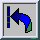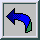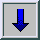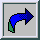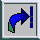"Scottish Book"

## 1  Manuscript and typescript

1. Copy of the manuscript of "Księga Szkocka" ("The Scottish Book") [PDF]  (6.6 MB) from the private archive of Stefan Banach's family.
It is available on this website with the written permission of Prof. Alina Banach - the owner of the manuscript.
2. English typescript of "The Scottish Book" [PDF]  (3.1 MB) from the Archive of the Library of Mathematics Faculty of Wrocław University.
It is available on this website with the written permission of Prof. Alina Banach and the Archive of the Mathematics Faculty Library of Wrocław University - the owner of the typescript.

## 2  List of Problems from the "Scottish Book"

1. Problem 1 by Banach (1935.07.17)
2. Problem 2 by Banach & Ulam
3. Problem 3 by Banach & Ulam
4. Problem 4 by Schreier
5. Problem 5 by Mazur
6. Problem 6 by Mazur & Orlicz [prize: a bottle of wine]
7. Problem 7 by Mazur & Banach
8. Problem 8 by Mazur [prize: five small beers]
9. Problem 9 by Mazur & Orlicz
10. Problem 10 by Banach & Mazur
11. Problem 10.1 by Mazur, Auerbach, Ulam & Banach
12. Problem 11 by Banach & Ulam
13. Problem 12 by Banach
14. Problem 13 by Ulam
15. Problem 14 by Schauder & Mazur
16. Problem 15 by Schauder
17. Problem 15.1 by Mazur & Orlicz [prize: two small beers]
18. Problem 16 by Ulam
19. Problem 17 by Ulam
20. Problem 17.1 by Ulam
21. Problem 18 by Ulam
22. Problem 19 by Ulam
23. Problem 20 by Ulam
24. Problem 20.1 by Mazur & Orlicz
25. Problem 21 by Ulam
26. Problem 22 by Ulam & Schreier
27. Problem 23 by Schauder
28. Problem 24 by Mazur [prize: two small beers]
29. Problem 25 by Schauder
30. Problem 26 by Mazur & Orlicz [prize: one small beer (Mazur)]
31. Problem 27 by Mazur & Orlicz [prize: five small beers (Mazur)]
32. Problem 28 by Mazur [prize: a bottle of wine]
33. Problem 29 by Ulam
34. Problem 30 by Ulam
35. Problem 31 by Ulam (1935.07.18)
36. Problem 32 by Ulam
37. Problem 33 by Ulam
38. Problem 34 by Ulam
39. Problem 35 by Ulam
40. Problem 36 by Ulam
41. Problem 37 by Ulam
42. Problem 38 by Ulam
43. Problem 39 by Auerbach
44. Problem 40 by Banach & Ulam (1935.07.26)
45. Problem 41 by Mazur
46. Problem 42 by Ulam
47. Problem 43 by Mazur [prize: a bottle of wine (Ulam)]
48. Problem 44 by Steinhaus
49. Problem 45 by Banach
50. Problem 46 by Banach
51. Problem 47 by Banach
52. Problem 48 by Mazur & Banach
53. Problem 49 by Mazur & Banach
54. Problem 50 by Banach
55. Problem 51 by Mazur
56. Problem 52 by Banach
57. Problem 53 by Banach
58. Problem 54 by Schauder
59. Problem 55 by Mazur
60. Problem 56 by Mazur & Orlicz
61. Problem 57 by Ruziewicz
62. Problem 58 by Ruziewicz
63. Problem 59 by Ruziewicz
64. Problem 60 by Ruziewicz
65. Problem 61 by Steinhaus
66. Problem 62 by Mazur & Ulam
67. Problem 63 by Mazur & Ulam
68. Problem 64 by Mazur
69. Problem 65 by Mazur
70. Problem 66 by Mazur
71. Problem 67 by Banach (1935.08.1)
72. Problem 68 by Ulam
73. Problem 69 by Mazur & Ulam
74. Problem 70 by Ulam
75. Problem 71 by Ulam
76. Problem 72 by Mazur
77. Problem 73 by Mazur & Orlicz
78. Problem 74 by Mazur & Orlicz
79. Problem 75 by Mazur
80. Problem 76 by Mazur
81. Problem 77 by Ulam [prize: a bottle of wine (Eilenberg)]
82. Problem 78 by Steinhaus (1935.08.2)
83. Problem 79 by Mazur & Orlicz
84. Problem 80 by Mazur
85. Problem 81 by Steinhaus (1935.08.6)
86. Problem 82 by Steinhaus (1935.08.6)
87. Problem 83 by Auerbach
88. Problem 84 by Auerbach
89. Problem 85 by Banach
90. Problem 86 by Banach
91. Problem 87 by Banach
92. Problem 88 by Mazur
93. Problem 89 by Mazur
94. Problem 90 by Ulam & Auerbach
95. Problem 91 by Mazur
96. Problem 92 by Mazur
97. Problem 93 by Mazur
98. Problem 94 by Łomnicki & Ulam
99. Problem 95 by Schreier & Ulam
100. Problem 96 by Ulam
101. Problem 97 by Kuratowski & Ulam
102. Problem 98 by Schreier & Ulam
103. Problem 99 by Ulam
104. Problem 100 by Ulam & Banach
105. Problem 101 by Ulam
106. Problem 102 by Ulam
107. Problem 103 by Schreier & Ulam
108. Problem 104 by Schauder
109. Problem 105 by Schauder
110. Problem 106 by Banach [prize: a bottle of wine]
111. Problem 107 by Sternbach
112. Problem 108 by Banach, Mazur & Ulam
113. Problem 109 by Mazur & Ulam (1935.10.16)
114. Problem 110 by Ulam [prize: a bottle of wine] (1935.10.1)
115. Problem 111 by Schreier
116. Problem 112 by Schreier
117. Problem 113 by Schreier
118. Problem 114 by Auerbach & Ulam
119. Problem 115 by Ulam
120. Problem 116 by Schreier & Ulam
121. Problem 117 by Fréchet (original manuscript in French)
122. Problem 118 by Fréchet (original manuscript in French)
123. Problem 119 by Orlicz
124. Problem 120 by Orlicz
125. Problem 121 by Orlicz
126. Problem 122 by Mazur & Orlicz
127. Problem 123 by Steinhaus
128. Problem 124 by Marcinkiewicz
129. Problem 125 by Infeld
130. Problem 126 by Kac
131. Problem 127 by Kuratowski
132. Problem 128 by Nikliborc
133. Problem 129 by Nikliborc
134. Problem 130 by Kaczmarz
135. Problem 131 by Zygmunt
136. Problem 132 by Sierpiński (1936.02.25)
137. Problem 133 by Eilenberg
138. Problem 134 by Eilenberg
139. Problem 135 by Eilenberg
140. Problem 136 by Eilenberg
141. Problem 137 by Eilenberg
142. Problem 138 by Eilenberg (1936.05.17)
143. Problem 139 by Ulam
144. Problem 140 by Ulam
145. Problem 141 by Ulam
146. Problem 142 by Ulam (theorem by Garrett & Birkhoff)
147. Problem 143 by Mazur
148. Problem 144 by Mazur & Ulam
149. Problem 145 by Ulam
150. Problem 146 by Ulam
151. Problem 147 by Auerbach & Mazur (1936.09.4)
152. Problem 148 by Auerbach
153. Problem 149 by Nikliborc
154. Problem 150 by Nikliborc
155. Problem 151 by Wavre (original manuscript in French) [prize: a fondue in Geneva] (1936.11.06)
156. Problem 152 by Steinhaus [prizes: (a) 100g of caviar, (b) a small beer, (c) a demitasse] (1936.11.06)
157. Problem 153 by Mazur [prize: a life goose] (1936.11.06)
158. Problem 154 by Mazur (1936.11.15)
159. Problem 155 by Mazur & Sternbach (1937.03.23)
160. Problem 156 by Ward (original manuscript in English) (1937.03.23)
161. Problem 157 by Ward (original manuscript in English) [prize: lunch at the "Dorothy" in Cambridge] (1937.03.23)
162. Problem 158 by Stoilow (original manuscript in French) (1937.05.1)
163. Problem 159 by Ruziewicz (1937.05.22)
164. Problem 160 by Mazur (1937.06.10)
165. Problem 161 by Kac
166. Problem 162 by Steinhaus [prize: dinner at "George's"] (1937.07.03)
167. Problem 163 by by von Neumann (original manuscript in German) [prize: a bottle of whisky of measure > 0] (1937.07.04)
168. Problem 164 by Ulam
169. Problem 165 by Ulam [prize: two bottles of wine]
170. Problem 166 by Ulam
171. Problem 167 by Ulam
172. Problem 168 by Ulam [prize: two bottles of beer]
173. Problem 169 by Szpilrajn
174. Problem 170 by Szpilrajn
175. Problem 171 by Schreier & Ulam
176. Problem 172 by Eidelheit (1938.06.04)
177. Problem 173 by Eidelheit
178. Problem 174 by Eidelheit (1938.06.23)
179. Problem 175 by Borsuk (1938.08.10)
180. Problem 176 by Eidelheit (1938.09.12)
181. Problem 177 by Kac (1938.09.11)
182. Problem 178 by Kac
183. Problem 179 by Offord (original manuscript in English) (1939.01.10)
184. Problem 180 by Kampe de Feriet (original manuscript in French) (1939.05.16)
185. Problem 181 by Steinhaus
186. Problem 182 by Knaster (1939.12.31) [prize: small light beer]
187. Problem 183 by Bogolubov (original manuscript in French) (1940.02.08)
188. Problem 184 by Saks [prize: one kilogram of bacon] (1940.02.08)
189. Problem 185 by Saks
190. Problem 186 by Banach (1940.03.21)
191. Problem 187 by Akexandroff (original manuscript in French) (1940.04.19)
192. Problem 188 by Sobolew (original manuscript in Russian and French) [prize: a bottle of wine] (1940.04.20)
193. Problem 188.1 by Eidelheit (1940.11.27)
194. Problem 189 by Fermant (original manuscript in Russian)
195. Problem 190 by Lusternik (original manuscript in Russian) [prize: a bottle of champagne] (1941.02.04)
196. Problem 191 by Szpilrajn (April 1941)
197. Problem 192 by Knaster & Szpilrajn (May 1941)
198. Problem 193 by Steinhaus (1941.05.31)

File translated from TEX by TTHgold, version 4.00.
On 04 Jan 2012, 18:51.